# Train Conditional Generative Adversarial Network (CGAN)

This example shows how to train a conditional generative adversarial network to generate images.

A generative adversarial network (GAN) is a type of deep learning network that can generate data with similar characteristics as the input training data.

A GAN consists of two networks that train together:

1. Generator — Given a vector of random values as input, this network generates data with the same structure as the training data.

2. Discriminator — Given batches of data containing observations from both the training data, and generated data from the generator, this network attempts to classify the observations as `"real"` or `"generated"`.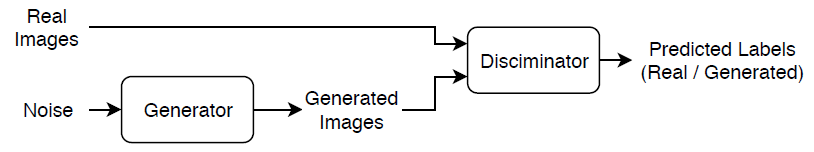A conditional generative adversarial network (CGAN) is a type of GAN that also takes advantage of labels during the training process.

1. Generator — Given a label and random array as input, this network generates data with the same structure as the training data observations corresponding to the same label.

2. Discriminator — Given batches of labeled data containing observations from both the training data and generated data from the generator, this network attempts to classify the observations as `"real"` or `"generated"`.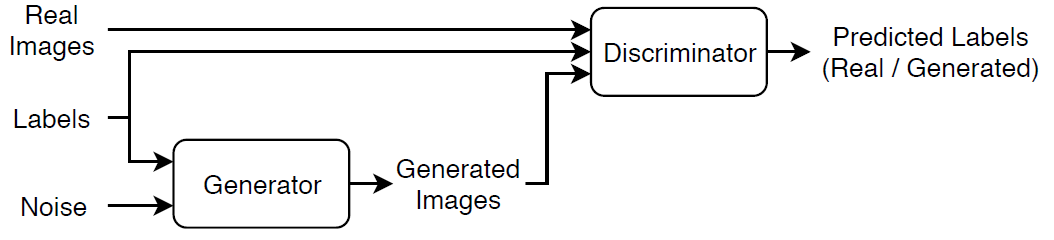To train a conditional GAN, train both networks simultaneously to maximize the performance of both:

• Train the generator to generate data that "fools" the discriminator.

• Train the discriminator to distinguish between real and generated data.

To maximize the performance of the generator, maximize the loss of the discriminator when given generated labeled data. That is, the objective of the generator is to generate labeled data that the discriminator classifies as `"real"`.

To maximize the performance of the discriminator, minimize the loss of the discriminator when given batches of both real and generated labeled data. That is, the objective of the discriminator is to not be "fooled" by the generator.

Ideally, these strategies result in a generator that generates convincingly realistic data that corresponds to the input labels and a discriminator that has learned strong feature representations that are characteristic of the training data for each label.

```url = "http://download.tensorflow.org/example_images/flower_photos.tgz"; downloadFolder = tempdir; filename = fullfile(downloadFolder,"flower_dataset.tgz"); imageFolder = fullfile(downloadFolder,"flower_photos"); if ~exist(imageFolder,"dir") disp("Downloading Flowers data set (218 MB)...") websave(filename,url); untar(filename,downloadFolder) end```

Create an image datastore containing the photos of the flowers.

```datasetFolder = fullfile(imageFolder); imds = imageDatastore(datasetFolder,IncludeSubfolders=true,LabelSource="foldernames");```

View the number of classes.

```classes = categories(imds.Labels); numClasses = numel(classes)```
```numClasses = 5 ```

Augment the data to include random horizontal flipping and resize the images to have size 64-by-64.

```augmenter = imageDataAugmenter(RandXReflection=true); augimds = augmentedImageDatastore([64 64],imds,DataAugmentation=augmenter);```

### Define Generator Network

Define the following two-input network, which generates images given random vectors of size 100 and corresponding labels.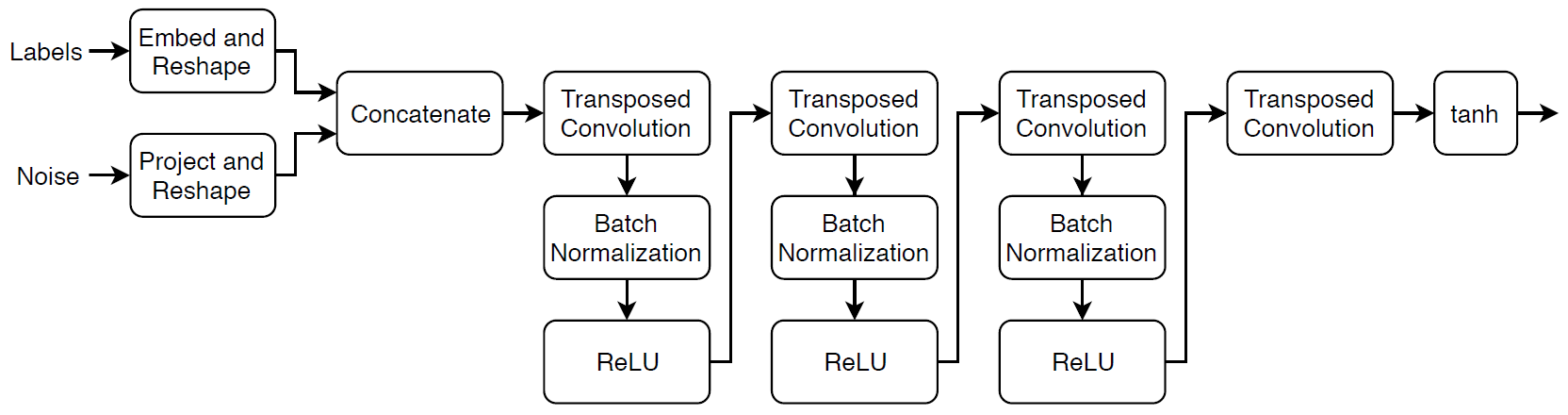This network:

• Converts the random vectors of size 100 to 4-by-4-by-1024 arrays using a fully connected layer followed by a reshape operation.

• Converts the categorical labels to embedding vectors and reshapes them to a 4-by-4 array.

• Concatenates the resulting images from the two inputs along the channel dimension. The output is a 4-by-4-by-1025 array.

• Upscales the resulting arrays to 64-by-64-by-3 arrays using a series of transposed convolution layers with batch normalization and ReLU layers.

Define this network architecture as a layer graph and specify the following network properties.

• For the categorical inputs, use an embedding dimension of 50.

• For the transposed convolution layers, specify 5-by-5 filters with a decreasing number of filters for each layer, a stride of 2, and `"same"` cropping of the output.

• For the final transposed convolution layer, specify a three 5-by-5 filter corresponding to the three RGB channels of the generated images.

• At the end of the network, include a tanh layer.

To project and reshape the noise input, use a fully connected layer followed by a reshape operation specified as a function layer with function given by the `feature2image` function, attached to this example as a supporting file. To embed the categorical labels, use the custom layer `embeddingLayer` attached to this example as a supporting file. To access these supporting files, open the example as a live script.

```numLatentInputs = 100; embeddingDimension = 50; numFilters = 64; filterSize = 5; projectionSize = [4 4 1024]; layersGenerator = [ featureInputLayer(numLatentInputs) fullyConnectedLayer(prod(projectionSize)) functionLayer(@(X) feature2image(X,projectionSize),Formattable=true) concatenationLayer(3,2,Name="cat"); transposedConv2dLayer(filterSize,4*numFilters) batchNormalizationLayer reluLayer transposedConv2dLayer(filterSize,2*numFilters,Stride=2,Cropping="same") batchNormalizationLayer reluLayer transposedConv2dLayer(filterSize,numFilters,Stride=2,Cropping="same") batchNormalizationLayer reluLayer transposedConv2dLayer(filterSize,3,Stride=2,Cropping="same") tanhLayer]; lgraphGenerator = layerGraph(layersGenerator); layers = [ featureInputLayer(1) embeddingLayer(embeddingDimension,numClasses) fullyConnectedLayer(prod(projectionSize(1:2))) functionLayer(@(X) feature2image(X,[projectionSize(1:2) 1]),Formattable=true,Name="emb_reshape")]; lgraphGenerator = addLayers(lgraphGenerator,layers); lgraphGenerator = connectLayers(lgraphGenerator,"emb_reshape","cat/in2");```

To train the network with a custom training loop and enable automatic differentiation, convert the layer graph to a `dlnetwork` object.

`netG = dlnetwork(lgraphGenerator)`
```dlnetGenerator = dlnetwork with properties: Layers: [19×1 nnet.cnn.layer.Layer] Connections: [18×2 table] Learnables: [19×3 table] State: [6×3 table] InputNames: {'input' 'input_1'} OutputNames: {'layer_2'} Initialized: 1 ```

### Define Discriminator Network

Define the following two-input network, which classifies real and generated 64-by-64 images given a set of images and the corresponding labels.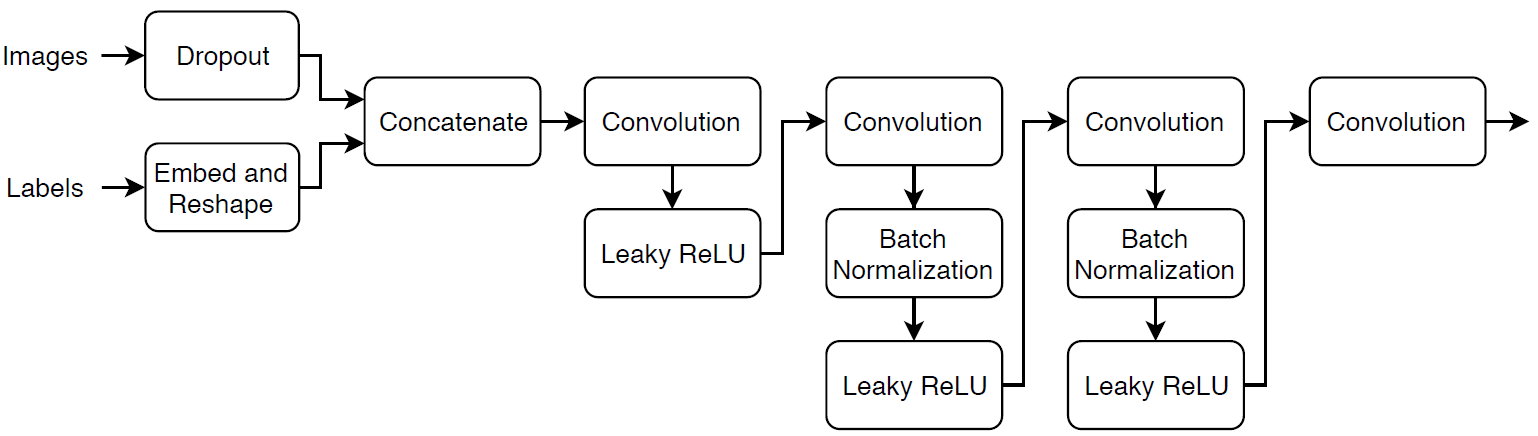Create a network that takes as input 64-by-64-by-1 images and the corresponding labels and outputs a scalar prediction score using a series of convolution layers with batch normalization and leaky ReLU layers. Add noise to the input images using dropout.

• For the dropout layer, specify a dropout probability of 0.75.

• For the convolution layers, specify 5-by-5 filters with an increasing number of filters for each layer. Also specify a stride of 2 and padding of the output on each edge.

• For the leaky ReLU layers, specify a scale of 0.2.

• For the final layer, specify a convolution layer with one 4-by-4 filter.

```dropoutProb = 0.75; numFilters = 64; scale = 0.2; inputSize = [64 64 3]; filterSize = 5; layersDiscriminator = [ imageInputLayer(inputSize,Normalization="none") dropoutLayer(dropoutProb) concatenationLayer(3,2,Name="cat") convolution2dLayer(filterSize,numFilters,Stride=2,Padding="same") leakyReluLayer(scale) convolution2dLayer(filterSize,2*numFilters,Stride=2,Padding="same") batchNormalizationLayer leakyReluLayer(scale) convolution2dLayer(filterSize,4*numFilters,Stride=2,Padding="same") batchNormalizationLayer leakyReluLayer(scale) convolution2dLayer(filterSize,8*numFilters,Stride=2,Padding="same") batchNormalizationLayer leakyReluLayer(scale) convolution2dLayer(4,1)]; lgraphDiscriminator = layerGraph(layersDiscriminator); layers = [ featureInputLayer(1) embeddingLayer(embeddingDimension,numClasses) fullyConnectedLayer(prod(inputSize(1:2))) functionLayer(@(X) feature2image(X,[inputSize(1:2) 1]),Formattable=true,Name="emb_reshape")]; lgraphDiscriminator = addLayers(lgraphDiscriminator,layers); lgraphDiscriminator = connectLayers(lgraphDiscriminator,"emb_reshape","cat/in2");```

To train the network with a custom training loop and enable automatic differentiation, convert the layer graph to a `dlnetwork` object.

`netD = dlnetwork(lgraphDiscriminator)`
```dlnetDiscriminator = dlnetwork with properties: Layers: [19×1 nnet.cnn.layer.Layer] Connections: [18×2 table] Learnables: [19×3 table] State: [6×3 table] InputNames: {'imageinput' 'input'} OutputNames: {'conv_5'} Initialized: 1 ```

### Define Model Loss Functions

Create the function `modelLoss`, listed in the Model Loss Function section of the example, which takes as input the generator and discriminator networks, a mini-batch of input data, and an array of random values, and returns the gradients of the loss with respect to the learnable parameters in the networks and an array of generated images.

### Specify Training Options

Train with a mini-batch size of 128 for 500 epochs.

```numEpochs = 500; miniBatchSize = 128;```

Specify the options for Adam optimization. For both networks, use:

• A learning rate of 0.0002

• A gradient decay factor of 0.5

• A squared gradient decay factor of 0.999

```learnRate = 0.0002; gradientDecayFactor = 0.5; squaredGradientDecayFactor = 0.999;```

Update the training progress plots every 100 iterations.

`validationFrequency = 100;`

If the discriminator learns to discriminate between real and generated images too quickly, then the generator can fail to train. To better balance the learning of the discriminator and the generator, randomly flip the labels of a proportion of the real images. Specify a flip factor of 0.5.

`flipFactor = 0.5;`

### Train Model

Train the model using a custom training loop. Loop over the training data and update the network parameters at each iteration. To monitor the training progress, display a batch of generated images using a held-out array of random values to input into the generator and the network scores.

Use `minibatchqueue` to process and manage the mini-batches of images during training. For each mini-batch:

• Use the custom mini-batch preprocessing function `preprocessMiniBatch` (defined at the end of this example) to rescale the images in the range `[-1,1]`.

• Discard any partial mini-batches with less than 128 observations.

• Format the image data with the dimension labels `"SSCB"` (spatial, spatial, channel, batch).

• Format the label data with the dimension labels `"BC"` (batch, channel).

• Train on a GPU if one is available. When the `OutputEnvironment` option of `minibatchqueue` is `"auto"`, `minibatchqueue` converts each output to a `gpuArray` if a GPU is available. Using a GPU requires Parallel Computing Toolbox™ and a supported GPU device. For information on supported devices, see GPU Computing Requirements (Parallel Computing Toolbox).

The `minibatchqueue` object, by default, converts the data to `dlarray` objects with underlying type `single`.

```augimds.MiniBatchSize = miniBatchSize; executionEnvironment = "auto"; mbq = minibatchqueue(augimds, ... MiniBatchSize=miniBatchSize, ... PartialMiniBatch="discard", ... MiniBatchFcn=@preprocessData, ... MiniBatchFormat=["SSCB" "BC"], ... OutputEnvironment=executionEnvironment); ```

Initialize the parameters for the Adam optimizer.

```velocityD = []; trailingAvgG = []; trailingAvgSqG = []; trailingAvgD = []; trailingAvgSqD = [];```

Initialize the plot of the training progress. Create a figure and resize it to have twice the width.

```f = figure; f.Position(3) = 2*f.Position(3);```

Create subplots of the generated images and of the scores plot.

```imageAxes = subplot(1,2,1); scoreAxes = subplot(1,2,2);```

Initialize animated lines for the scores plot.

```lineScoreGenerator = animatedline(scoreAxes,Color=[0 0.447 0.741]); lineScoreDiscriminator = animatedline(scoreAxes,Color=[0.85 0.325 0.098]);```

Customize the appearance of the plots.

```legend("Generator","Discriminator"); ylim([0 1]) xlabel("Iteration") ylabel("Score") grid on```

To monitor training progress, create a held-out batch of 25 random vectors and a corresponding set of labels 1 through 5 (corresponding to the classes) repeated five times.

```numValidationImagesPerClass = 5; ZValidation = randn(numLatentInputs,numValidationImagesPerClass*numClasses,"single"); TValidation = single(repmat(1:numClasses,[1 numValidationImagesPerClass]));```

Convert the data to `dlarray` objects and specify the dimension labels `"CB"` (channel, batch).

```ZValidation = dlarray(ZValidation,"CB"); TValidation = dlarray(TValidation,"CB");```

For GPU training, convert the data to `gpuArray` objects.

```if (executionEnvironment == "auto" && canUseGPU) || executionEnvironment == "gpu" ZValidation = gpuArray(ZValidation); TValidation = gpuArray(TValidation); end```

Train the conditional GAN. For each epoch, shuffle the data and loop over mini-batches of data.

For each mini-batch:

• Evaluate the gradients of the loss with respect to the learnable parameters, the generator state, and the network scores using `dlfeval` and the `modelLoss` function.

• Update the network parameters using the `adamupdate` function.

• Plot the scores of the two networks.

• After every `validationFrequency` iterations, display a batch of generated images for a fixed held-out generator input.

Training can take some time to run.

```iteration = 0; start = tic; % Loop over epochs. for epoch = 1:numEpochs % Reset and shuffle data. shuffle(mbq); % Loop over mini-batches. while hasdata(mbq) iteration = iteration + 1; % Read mini-batch of data. [X,T] = next(mbq); % Generate latent inputs for the generator network. Convert to % dlarray and specify the dimension labels "CB" (channel, batch). % If training on a GPU, then convert latent inputs to gpuArray. Z = randn(numLatentInputs,miniBatchSize,"single"); Z = dlarray(Z,"CB"); if (executionEnvironment == "auto" && canUseGPU) || executionEnvironment == "gpu" Z = gpuArray(Z); end % Evaluate the gradients of the loss with respect to the learnable % parameters, the generator state, and the network scores using % dlfeval and the modelLoss function. [~,~,gradientsG,gradientsD,stateG,scoreG,scoreD] = ... dlfeval(@modelLoss,netG,netD,X,T,Z,flipFactor); netG.State = stateG; % Update the discriminator network parameters. [netD,trailingAvgD,trailingAvgSqD] = adamupdate(netD, gradientsD, ... trailingAvgD, trailingAvgSqD, iteration, ... learnRate, gradientDecayFactor, squaredGradientDecayFactor); % Update the generator network parameters. [netG,trailingAvgG,trailingAvgSqG] = ... adamupdate(netG, gradientsG, ... trailingAvgG, trailingAvgSqG, iteration, ... learnRate, gradientDecayFactor, squaredGradientDecayFactor); % Every validationFrequency iterations, display batch of generated images using the % held-out generator input. if mod(iteration,validationFrequency) == 0 || iteration == 1 % Generate images using the held-out generator input. XGeneratedValidation = predict(netG,ZValidation,TValidation); % Tile and rescale the images in the range [0 1]. I = imtile(extractdata(XGeneratedValidation), ... GridSize=[numValidationImagesPerClass numClasses]); I = rescale(I); % Display the images. subplot(1,2,1); image(imageAxes,I) xticklabels([]); yticklabels([]); title("Generated Images"); end % Update the scores plot. subplot(1,2,2) addpoints(lineScoreGenerator,iteration,double(scoreG)); addpoints(lineScoreDiscriminator,iteration,double(scoreD)); % Update the title with training progress information. D = duration(0,0,toc(start),Format="hh:mm:ss"); title(... "Epoch: " + epoch + ", " + ... "Iteration: " + iteration + ", " + ... "Elapsed: " + string(D)) drawnow end end```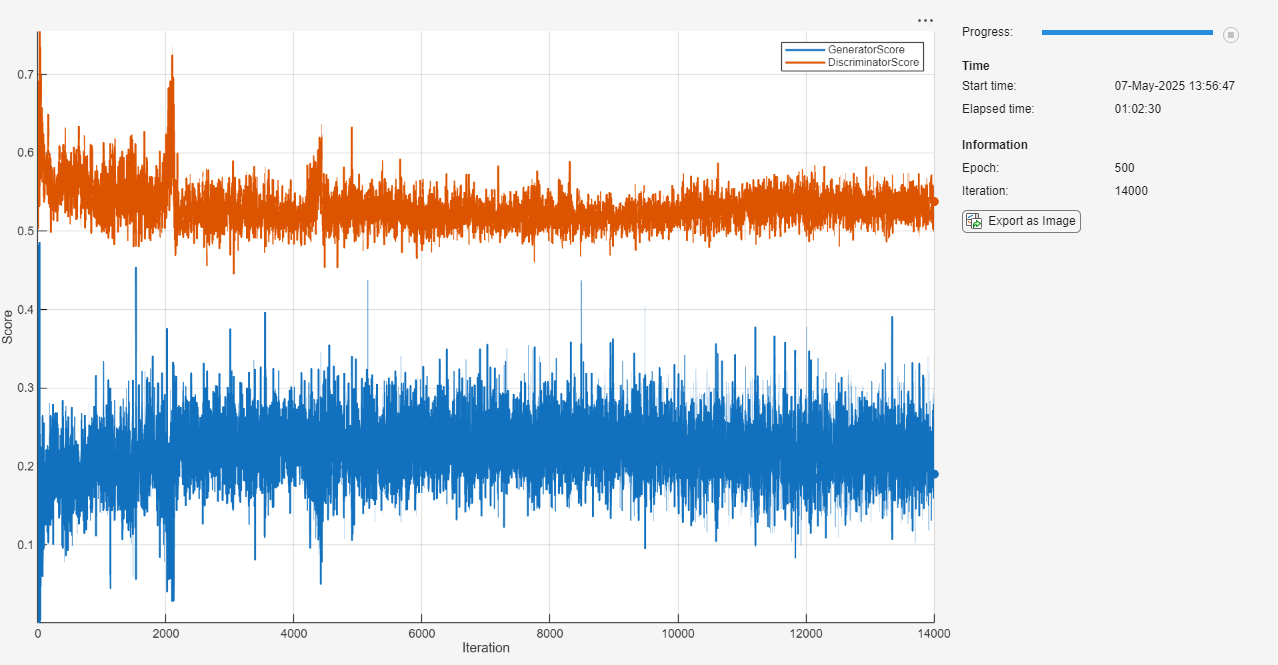Here, the discriminator has learned a strong feature representation that identifies real images among generated images. In turn, the generator has learned a similarly strong feature representation that allows it to generate images similar to the training data. Each column corresponds to a single class.

The training plot shows the scores of the generator and discriminator networks. To learn more about how to interpret the network scores, see Monitor GAN Training Progress and Identify Common Failure Modes.

### Generate New Images

To generate new images of a particular class, use the `predict` function on the generator with a `dlarray` object containing a batch of random vectors and an array of labels corresponding to the desired classes. Convert the data to `dlarray` objects and specify the dimension labels `"CB"` (channel, batch). For GPU prediction, convert the data to `gpuArray` objects. To display the images together, use the `imtile` function and rescale the images using the `rescale` function.

Create an array of 36 vectors of random values corresponding to the first class.

```numObservationsNew = 36; idxClass = 1; ZNew = randn(numLatentInputs,numObservationsNew,"single"); TNew = repmat(single(idxClass),[1 numObservationsNew]);```

Convert the data to `dlarray` objects with the dimension labels `"SSCB"` (spatial, spatial, channels, batch).

```ZNew = dlarray(ZNew,"CB"); TNew = dlarray(TNew,"CB");```

To generate images using the GPU, also convert the data to `gpuArray` objects.

```if (executionEnvironment == "auto" && canUseGPU) || executionEnvironment == "gpu" ZNew = gpuArray(ZNew); TNew = gpuArray(TNew); end```

Generate images using the `predict` function with the generator network.

`XGeneratedNew = predict(netG,ZNew,TNew);`

Display the generated images in a plot.

```figure I = imtile(extractdata(XGeneratedNew)); I = rescale(I); imshow(I) title("Class: " + classes(idxClass))```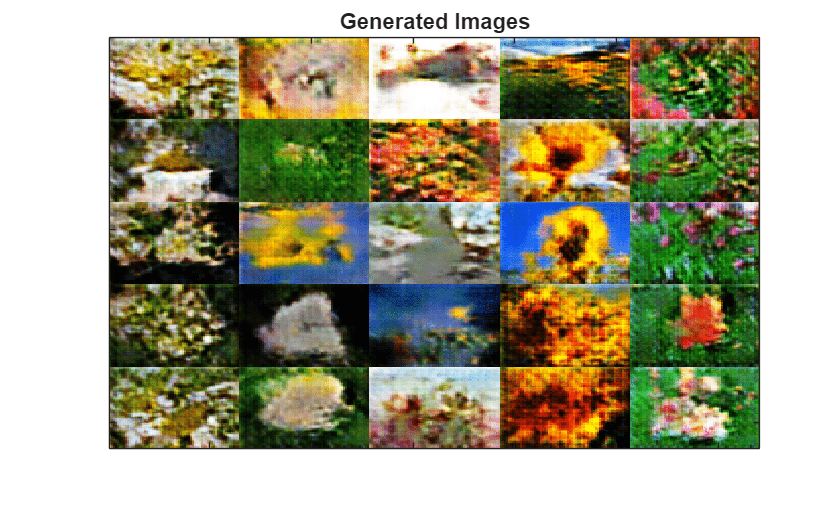Here, the generator network generates images conditioned on the specified class.

### Model Loss Function

The function `modelLoss` takes as input the generator and discriminator `dlnetwork` objects `netG` and `netD`, a mini-batch of input data `X`, the corresponding labels `T`, and an array of random values `Z`, and returns the gradients of the loss with respect to the learnable parameters in the networks, the generator state, and the network scores.

If the discriminator learns to discriminate between real and generated images too quickly, then the generator can fail to train. To better balance the learning of the discriminator and the generator, randomly flip the labels of a proportion of the real images.

```function [lossG,lossD,gradientsG,gradientsD,stateG,scoreG,scoreD] = ... modelLoss(netG,netD,X,T,Z,flipFactor) % Calculate the predictions for real data with the discriminator network. YReal = forward(netD,X,T); % Calculate the predictions for generated data with the discriminator network. [XGenerated,stateG] = forward(netG,Z,T); YGenerated = forward(netD,XGenerated,T); % Calculate probabilities. probGenerated = sigmoid(YGenerated); probReal = sigmoid(YReal); % Calculate the generator and discriminator scores. scoreG = mean(probGenerated); scoreD = (mean(probReal) + mean(1-probGenerated)) / 2; % Flip labels. numObservations = size(YReal,4); idx = randperm(numObservations,floor(flipFactor * numObservations)); probReal(:,:,:,idx) = 1 - probReal(:,:,:,idx); % Calculate the GAN loss. [lossG, lossD] = ganLoss(probReal,probGenerated); % For each network, calculate the gradients with respect to the loss. gradientsG = dlgradient(lossG,netG.Learnables,RetainData=true); gradientsD = dlgradient(lossD,netD.Learnables); end```

### GAN Loss Function

The objective of the generator is to generate data that the discriminator classifies as `"real"`. To maximize the probability that images from the generator are classified as real by the discriminator, minimize the negative log likelihood function.

Given the output $\mathit{Y}$ of the discriminator:

• $\stackrel{ˆ}{\mathit{Y}}=\sigma \left(\mathit{Y}\right)$ is the probability that the input image belongs to the class `"real"`.

• $1-\stackrel{ˆ}{\mathit{Y}}$ is the probability that the input image belongs to the class `"generated"`.

Note the sigmoid operation $\sigma$ happens in the `modelLoss` function. The loss function for the generator is given by

`$\mathrm{lossGenerator}=-\mathrm{mean}\left(\mathrm{log}\left({\stackrel{ˆ}{\mathit{Y}}}_{\mathrm{Generated}}\right)\right),$`

where ${\underset{}{\overset{ˆ}{Y}}}_{Generated}$ contains the discriminator output probabilities for the generated images.

The objective of the discriminator is to not be "fooled" by the generator. To maximize the probability that the discriminator successfully discriminates between the real and generated images, minimize the sum of the corresponding negative log likelihood functions. The loss function for the discriminator is given by

`$\mathrm{lossDiscriminator}=-\mathrm{mean}\left(\mathrm{log}\left({\stackrel{ˆ}{\mathit{Y}}}_{\mathrm{Real}}\right)\right)-\mathrm{mean}\left(\mathrm{log}\left(1-{\stackrel{ˆ}{\mathit{Y}}}_{\mathrm{Generated}}\right)\right),$`

where ${\underset{}{\overset{ˆ}{Y}}}_{Real}$ contains the discriminator output probabilities for the real images.

```function [lossG, lossD] = ganLoss(scoresReal,scoresGenerated) % Calculate losses for the discriminator network. lossGenerated = -mean(log(1 - scoresGenerated)); lossReal = -mean(log(scoresReal)); % Combine the losses for the discriminator network. lossD = lossReal + lossGenerated; % Calculate the loss for the generator network. lossG = -mean(log(scoresGenerated)); end```

### Mini-Batch Preprocessing Function

The `preprocessMiniBatch` function preprocesses the data using the following steps:

1. Extract the image and label data from the input cell arrays and concatenate them into numeric arrays.

2. Rescale the images to be in the range `[-1,1]`.

```function [X,T] = preprocessData(XCell,TCell) % Extract image data from cell and concatenate X = cat(4,XCell{:}); % Extract label data from cell and concatenate T = cat(1,TCell{:}); % Rescale the images in the range [-1 1]. X = rescale(X,-1,1,InputMin=0,InputMax=255); end```